Open in App
Not now

# Magnetic Field

• Last Updated : 28 Apr, 2021

Static Electric charges create Electric Field and if the Charges start to move, they create a Magnetic field. The force field is also developed by the Magnet, a permanent magnet that creates force known as the magnetic force that makes magnetic lines which are not visible by naked eyes, but certain experiments show that a magnet creates magnetic lines around them, For instance, Put a magnet under a sheet of paper and put iron filing on top of the piece of paper, it will be observed that the iron filing has created a pattern on the piece of paper, the pattern looks similar to the electric field lines, however, in magnetic field lines, the lines form a closed loop, Outside the magnet, they travel from north pole to south pole and inside the magnet, they travel from the south pole to the North Pole.

### Magnets and Magnetic fields

A Magnet is a material that has the ability to produce Magnetic force which creates magnetic field lines, the field force is able to attract and repel other ferromagnetic materials like iron, nickel, etc.

Magnetic field is a vector quantity (it has both magnitude and direction), the direction of the magnetic field is from the north pole to the South Pole. The Magnetic field lines always form closed curves, and the strength is determined by how close magnetic field lines are, the field lines are closer to each other when they are closer to the magnetic material and are away from each other when they are away from the material.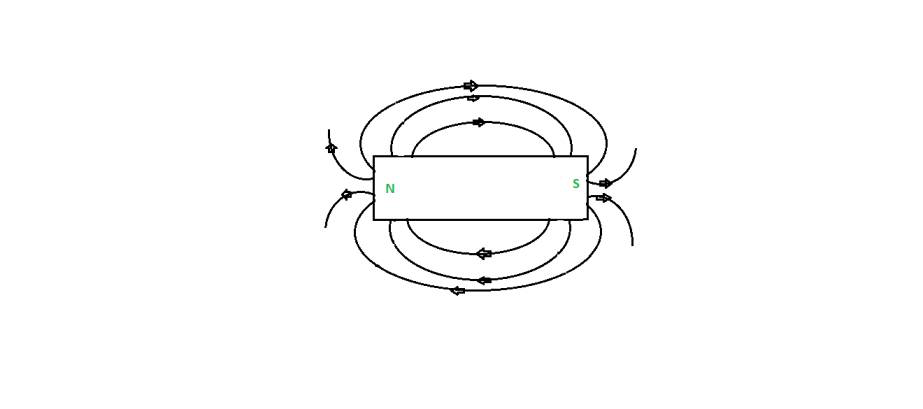### Magnetic effect of Electric Current

When current is passed through a magnetic material, a magnetic field is produced, if a needle is placed near the material, on providing the electric charge (moving charges), the needle will deflect. The area to the point where the force is exerted by the magnetic material is called a magnetic field.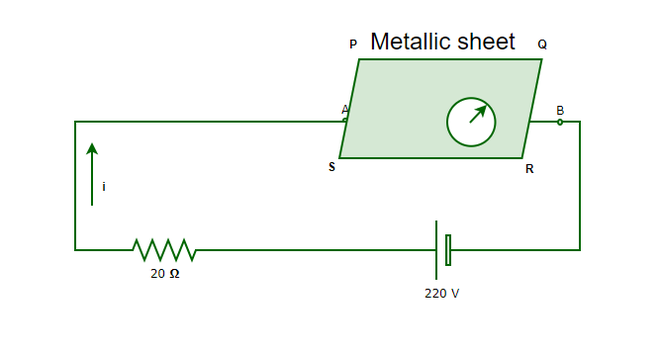Properties of Magnetic Field:

• The strength of the magnetic field is directly proportional to the density of the field lines produced by them.
• Magnetic field lines never cross each other.
• Magnetic fields will always have closed and continuous loops.

### Magnetic Field lines due to Current Carrying Straight Conductor

The magnetic field lines produced by a straight current carrying conductor is in the form of concentric circles, depending upon the direction of current, magnetic field lines also change their directions. The direction of the magnetic field lines can be identified by Right-hand Thumb Rule or Maxwell’s corkscrew rule.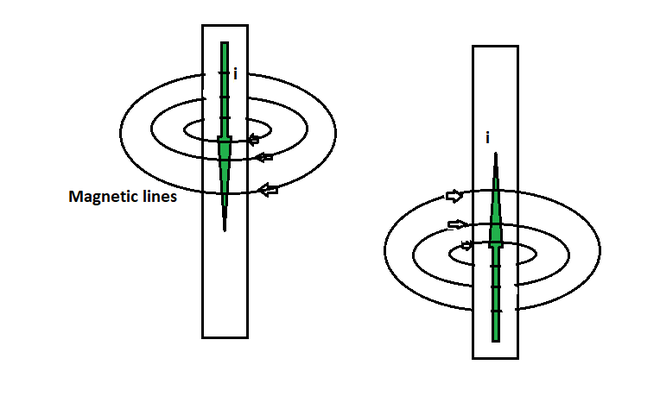Concentric Magnetic field lines by straight conductor carrying current

Right- Hand Thumb Rule:

According to Right- Hand Thumb Rule, If the right hand’s thumb is placed in the direction of the moving current, then the direction of fingers show the direction of magnetic field lines, Similarly, if the thumb is placed in the direction of the magnetic field lines, then the direction of current is shown by the curled fingers’ direction.

Maxwell’s Corkscrew Rule:

According to Maxwell’s Corkscrew rule, A screw can tell the direction of the magnetic field lines. If the screw is moved forward, the direction of rotation shows the direction of magnetic field lines while the direction of the movement of the screw shows the direction of current and Vice-Versa.

### Magnetic Field lines due to Current through a Circular Loop

The magnetic field lines will create the same pattern as it created in the case of a straight current-carrying conductor, That means, the field lines will be concentric in nature, but based on the direction of the current, the direction of field lines will change as well. For example, in a circular loop, imagine two places, one where the current goes upward and one where the current goes downward, according to the right-hand thumb rule, in the first case, the field lines must be anticlockwise in nature, and in the second case, the field lines must be clockwise in nature.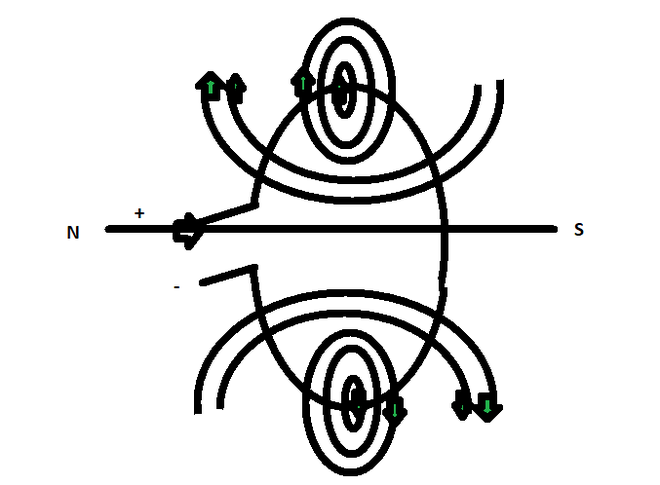### Magnetic Field lines due to Current in Solenoid

A solenoid is a helical coil which upon giving current becomes an electromagnet and forms North and South Pole and due to moving charges, it creates Magnetic field lines. The field lines created by the solenoid look similar to the field lines created by a simple Bar magnet.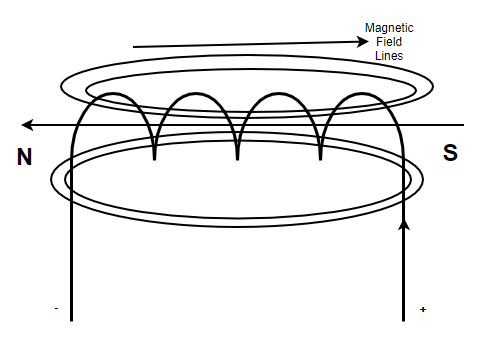### Electromagnet

An electromagnet is a type of magnet formed due to the current moving and passing through it, it is a type of temporary magnet and is mostly seen as a coil wrapped over a soft iron core (example, solenoid).

Properties of electromagnet:

• The electromagnets are not permanent magnets, they are temporary in nature.
• The strength of the magnetic force can be altered by varying current.
• Electromagnets are made with soft iron core.

Note: When current is applied on a ferromagnetic material to produce magnetic field lines, the magnetic force is dependent on the number of magnetic dipole aligned, The amount of current applied is directly proportional to the number of dipoles alignment and the amount of magnetic force can be altered. However, it can only be done up to some point, till the point where saturation reaches and no dipoles can further be available for alignment.

### Force on a current carrying conductor placed in a Magnetic Field

Imagine a wire (conductor) is placed under a magnet and current is passed through the wire, due to the moving electric charges in a magnetic field, a force is applied to the conductor that moves it in a certain direction, this phenomenon was given by Michael faraday. The direction of the force on conductor is determined by Fleming’s left-hand rule.

Fleming’s Left-hand Rule:

According to Fleming’s left-hand rule, the direction of force can be determined with the help of left hand’s thumb, index finger and middle finger. The thumb represents the Force, The index finger shows the direction of the magnetic field lines and the middle finger shows the direction of current. Therefore, if the middle finger and index finger is kept in the direction of current and magnetic field lines respectively, the thumb will represent the direction of force produced on current carrying conductor.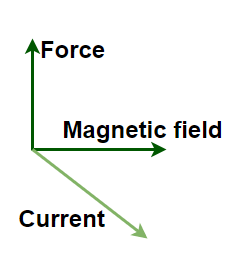### Sample Questions

Question 1: Why does Magnetic field lines never intersect each other?

It is one of the properties of Magnetic field lines that they never intersect each other, if they do so, the intersection point of magnetic field lines will show two different directions which is not possible for the field lines as they follow one direction.

Question 2: Why does magnetic field lines vary in density?

Magnetic field lines can vary in density because the field lines start near the magnet, and then they go further away and as they go further away from the magnet, the effect of magnetic force gradually decreases and stops after a point, since the force is decreasing, the density of the magnetic field lines also tend to decrease.

Question 3: What are the applications of Fleming’s left-hand rule?

Fleming’s left-hand rule is applied in Electric motors (DC motors, induction motors, etc). The basic concept is that the induced current produces force on the conductor which helps in creating motion.

Question 4: What is the difference between Permanent magnet and electromagnet?

A Permanent magnet is made up of Hard material, The magnetic field is produced due to magnetization and once the magnetization is lost, the magnet ceases to show magnetic properties since the magnetic dipoles cannot be changed further.

An electromagnet is made up of Soft material, The magnetic field is produced by applying current through it. Once the current stops, the magnetism stops but again develops after applying current again, The dipoles aligned in an electromagnet can be altered again and again.

Question 5: Write down the Properties of Magnetic field lines.